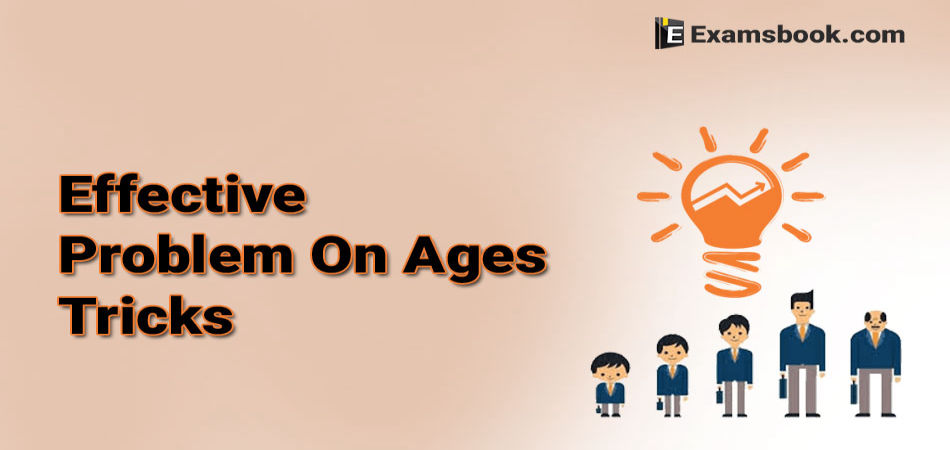• Save

# Effective Problem On Ages Tricks For Bank And SSC Exams

Last year 2.4K ViewsMathematics is a complex subject for those students are preparing for SSC and Banking exams. Problem ages is an important topic of this subject. In this topic, we get two and more person ages and ratios or relation between their age.

To solve these questions, we have a very short time in the exam. In this blog, I am given some questions to practice and along with a short trick to solve them effectively with speed. By using these tricks you can save your important time during the exams.

As well as these tricks, you can visit problems on ages with solutions to know how to solve these type of questions.

## Problem On Ages Tricks with Solution for Competitive Exams:

Example 1: Present age of Sameer and Anand are in the ratio of 5: 4 respectively. Three years hence, the ratio of their ages will become 11: 9 respectively. What is Anand’s present age in years?

(A) 24

(B) 27

(C) 40

(D) None of these

Solution:

In this question, we have to get Anand present age.

The ratio of Sameer and Anand is 5: 4.

Short trick:

We have to divide all the options by the ratio of Anand = 4.

Option (A) and (C) values 24 and 27 are fully divided by ratio of Anand.

Next,

Statement is Three years hence, the ratio of their ages will become 11: 9.

So, we have to add 3 year in Option (A) and Option (C).

Then the option (A) and option (C) Values are

= 24+3 year = 27 years,

= 40+3 years = 43 years,

Next,

Option (A) and (C) are divided of ratio of Anand = 9

Option (A) is fully divided by ratio of Anand 9.

Example 2:  Present age of A and B are in the ratio of 5: 6 respectively. Seven years hence this ratio will become 6: 7 respectively. What is A’s present age in years?

(A) 35

(B) 42

(C) 49

(D) None of these

Solution.

In this question, we have to get A present age.

The ratio of A and B is 5: 6.

Short trick

We have to divide all the options by the ratio of A = 5.

Option (A) value 35 is fully divided by ratio of A.

Next,

Statement is Seven years hence; the ratio of their ages will become 6: 7.

So, we have to added 7 year in Option and Option (A).

Then the option (A) Value is

= 35+7 year = 42 years,

Next,

Option (A) is divided of ratio of A = 6

Option (A) is fully divided by ratio of Anand 6.

Example 3: The age of two persons differ by 16 years. If 6 years ago, the elder one be 3 times as old as the younger one, find their present ages.

Solution

Let the age of the younger person be X years.

Then, age of the elder person = (x+16) years.

⸫ 3(X -16) = (X+16-6) ↔ 3X – 18

= X+10 ↔2X = 28 ↔ X=14.

Hence, their present age are 14 years and 30 years.

Example 4: The present age of a father is 3 years more than three times the age of his son. Three years hence, father’s age will be 10 years more than twice the age of the son. Find the present age of the father.

Solution

⸫ Let the son’s present age be x years. Then, Father’s present age = (3X+3) years.

⸫ (3x+3+3) = 2(x+3)+10

↔ 3X+6) = (3×10+3) years = 33 years.

### Problem On Ages Practice Question:

Q.1. Madhav is younger than Mohan by 4 years. If their ages are in the respectively ratio of 7 : 9, how old is Madhav?

(A) 16.5 years

(B) 18 years

(C) 19.5 years

(D) 24.5 years

Ans .  D

Q.2. The ratio between the present age of Ram and Shyam is 6 : 7. If Shyam is 4 years old than Ram, what will be the ratio of the ages of Ram and Shyam after 4 years?

(A) 7 : 8

(B) 9 : 7

(C) 7 : 6

(D) 8 : 5

Ans .  A

Q.3. Six years ago, the ratio of the ages Salman and Sharukh Was 6 : 5. Four years hence, the ratio of their ages will be 11 : 10. What is Sharukh’s age at present ?

(A) 16 years

(B) 19 years

(C) 20 years

(D) 14 years.

Ans .  A

Q.4. The total of the ages of Mayank, Lucky and Naman is 93 years. Ten years ago, the ratio of their age was 2 : 3 : 4. What is the present age of Naman?

(A) 30 years

(B) 35 Years

(C) 34 Years

(D) 38 Years

Ans .  D

Q.5. Hansraj is 40 Year old and Ronnie is 60 years old. How many years ago was the ratio of their ages 3 : 5 ?

(A) 5 Years

(B) 10 Years

(C) 25 Years

(D) 35 Years

Ans .  B

If you want to practice more questions related to Problem on Ages then you can go to the link provided below.

In this post I have made every effort to explain the Problem on Ages in a simple way, even if you are having problems in any manner or question, you can Ask me by writing in the comment box below.